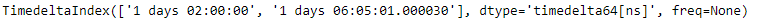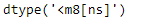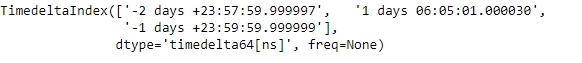Open in App
Not now

# Python | Pandas TimedeltaIndex.dtype

• Last Updated : 29 Dec, 2018

Python is a great language for doing data analysis, primarily because of the fantastic ecosystem of data-centric python packages. Pandas is one of those packages and makes importing and analyzing data much easier.

Pandas` TimedeltaIndex.dtype` attribute return the data type of the TimedeltaIndex object.

Syntax: TimedeltaIndex.dtype

Return : dtype

Example #1: Use `TimedeltaIndex.dtype` attribute to find the data type of the given TimedeltaIndex object.

 `# importing pandas as pd``import` `pandas as pd`` ` `# Create the TimedeltaIndex object``tidx ``=` `pd.TimedeltaIndex(data ``=``[``'1 days 02:00:00'``, ``                        ``'1 days 06:05:01.000030'``])`` ` `# Print the TimedeltaIndex``print``(tidx)`

Output :Now we want to find out the data type of the given TimedeltaIndex object.

 `# return the data type of the ``# values in the given object``tidx.dtype`

Output :As we can see in the output, the `TimedeltaIndex.dtype` has returned the underlying data type of the values in the tidx object.

Example #2: Use `TimedeltaIndex.dtype` attribute to find the data type of the given TimedeltaIndex object.

 `# importing pandas as pd``import` `pandas as pd`` ` `# Create the TimedeltaIndex object``tidx ``=` `pd.TimedeltaIndex(data ``=``[``'-1 days 2 min 3us'``, ``'1 days 06:05:01.000030'``,``                                                ``'-1 days + 23:59:59.999999'``])`` ` `# Print the TimedeltaIndex``print``(tidx)`

Output :Now we want to find out the data type of the given TimedeltaIndex object.

 `# return the data type of the``#  values in the given object``tidx.dtype`

Output :As we can see in the output, the `TimedeltaIndex.dtype` has returned the underlying data type of the values in the tidx object.

My Personal Notes arrow_drop_up